# Application Of Integrals NCERT Solutions : Class 12 Maths

## NCERT Solutions for Class 12 Maths : Application Of IntegralsFilter
Filters :
Classes
•
•
•
•
•
•
•
Chapters
•
•
•
•
•
•
•
•
•
•
• 3 More
Exercises
•
•
•
•

### NCERT Class 12 | APPLICATION OF INTEGRALS | Miscellaneous Exercise | Question No. 11

Using the method of integration find the area bounded by the curve |x|+|y|" "=" "1 . [Hint: The required region is bounded by lines x" "+" "y" "=" "1," "x " "-y" "=" "1," "" "-x" "+" "y" "=" "1 and " "-x" "" "-y" "=" "1]dotDoubtnut one of the best online education platform provides free NCERT Solutions of Maths for Class 12 Application Of Integrals which are solved by our maths experts as per the NCERT (CBSE) guidelines. We provide the solutions as video solutions in which concepts are also explained along with answer which will help students while learning , preparing for exams and in doing homework. These Solutions will help to revise complete syllabus and score more marks in examinations.

Get here free, accurate and comprehensive NCERT Solutions for Class 12 Maths Application Of Integrals which have been reviewed by our maths counsellors as per the latest edition following up the CBSE guidelines. We provide video solutions in which solutions to all the questions of NCERT Class 12 Maths textbook are explained in step by step and detailed way.

## The Topics of the Chapter Application Of Integrals are :

AREA BETWEEN TWO CURVES

AREA CALCULATION USING HORIZONTAL STRIPS

AREA CALCULATION USING VERTICAL STRIPS

AREA CALCULATION USING VERTICAL OR HORIZONTAL STRIPS

MISCELLANEOUS

It contains these exercises along with solved examples. All the exercises are solved in the video. Select the exercise to view the solutions exercisewise of the Chapter Application Of Integrals:

## NCERT Solutions Class 12 Maths Chapter Application Of Integrals Exercises:

We have covered all the exercises and also Solved examples in the videos. Along with the practise exercise Students should also practise solved examples to clear the concepts of the Application Of Integrals. If incase you have any doubt you can watch the solutions for the given questions.Watch the solutions for the given questions in which it is also explained in the video steps to solve the questions along with answers.

Until now, students must have been clear about concepts taught in the previous chapter ‘Integrals’ including all the topics and sub-topics, which is very necessary to understand the topic ‘Application of Integrals’. This chapter basically deals with the area of curves given by Cartesian Equations, and the area bounded by two or more curves. It is an important chapter of integral calculus as it has numerous applications throughout mathematics as well as in other subjects like physics. Here, at Doubtnut, we will be explaining the entire chapter in the form of topics and sub-topics for the easy and quick understanding of the students. On our website, doubtnut.com, we follow the exact pattern given in the NCERT textbook as it is the best and the most preferred content in the domain of school education under CBSE educational board. Students should give prime significance to this book irrespective of the others available in the market because it is included by CBSE in its curriculum; therefore, the NCERT book covers entire topics as per CBSE syllabus.  At Doubtnut, we follow a specific pattern for the better and progressive learning of the students. The solutions provided by doubtnut are curated and reviewed by the expert teachers who are well versed and skilled in their subjects. The subject matter experts have emphasize on topics that are important from the exam point of view.

However, there are a number of books and content available in the market and being circulated under different publication and authors, but students should not be confused to choose among the different materials, especially CBSE students, as NCERT is of supremely important for them as the book covers the core syllabus. Students should practice the questions given in NCERT regularly and trust it for their examinations, be it at the school level or national level, as more or less the questions have their reference from NCERT textbook. If a student regularly goes through every question and exercises, reads the summary periodically and revises the syllabus given in NCERT book, he/she would definitely not find any difficulty in any examination and even solving the questions of the other textbook.

For more, students should visit our website, Doubtnut.com, for best solutions of NCERT class 12 maths and top-notch explanation on each and every topic and questions related to it. On our website, we make sure that no topic is left unexplained and even we provide video lectures that are conducted by the best teachers who have deep knowledge about the subject, chapter, topics, etc. and they also know its importance in the examinations and its weight in the question paper. Students should reach out to us in any of the platforms for the best and authentic material.

## Topics & Subtopics of NCERT Class 12 Maths Chapter 8 – Application of Integrals

### Intregration

The mathematical concept of Integration can be apllied to different subjects like science and engineering. In this chapter, we will find ways and means to find the areas of the bounded regions.

### Areas of Curves

We can find the area under a curve between two points by doing a definite integral between the two points. For instance to find the area under the curve say, y=f(x) between x=a and x= b, integrate y= f(x) between the limits of a and b.

Area under the x- axis = negative

Area under the y- axis = positive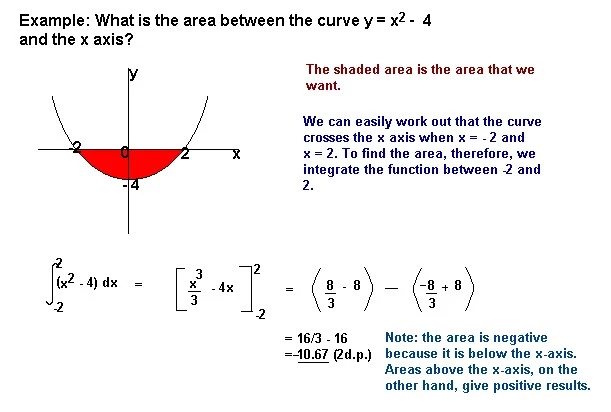Suppose that f(x) < 0 on some [a, b]. Then the area under the curve y=f(x) from x=a to x=b will be negative in sign, that is -

ab f(x) dx0

Once we consider how the definite integrals arrived at in the first place, as a limit of the sum of the n rectangles n. Thus, if f(x) < 0 in some intervals then the area of the triangle in the interval will also be negative.

This property means for example, if f(x) has the following form: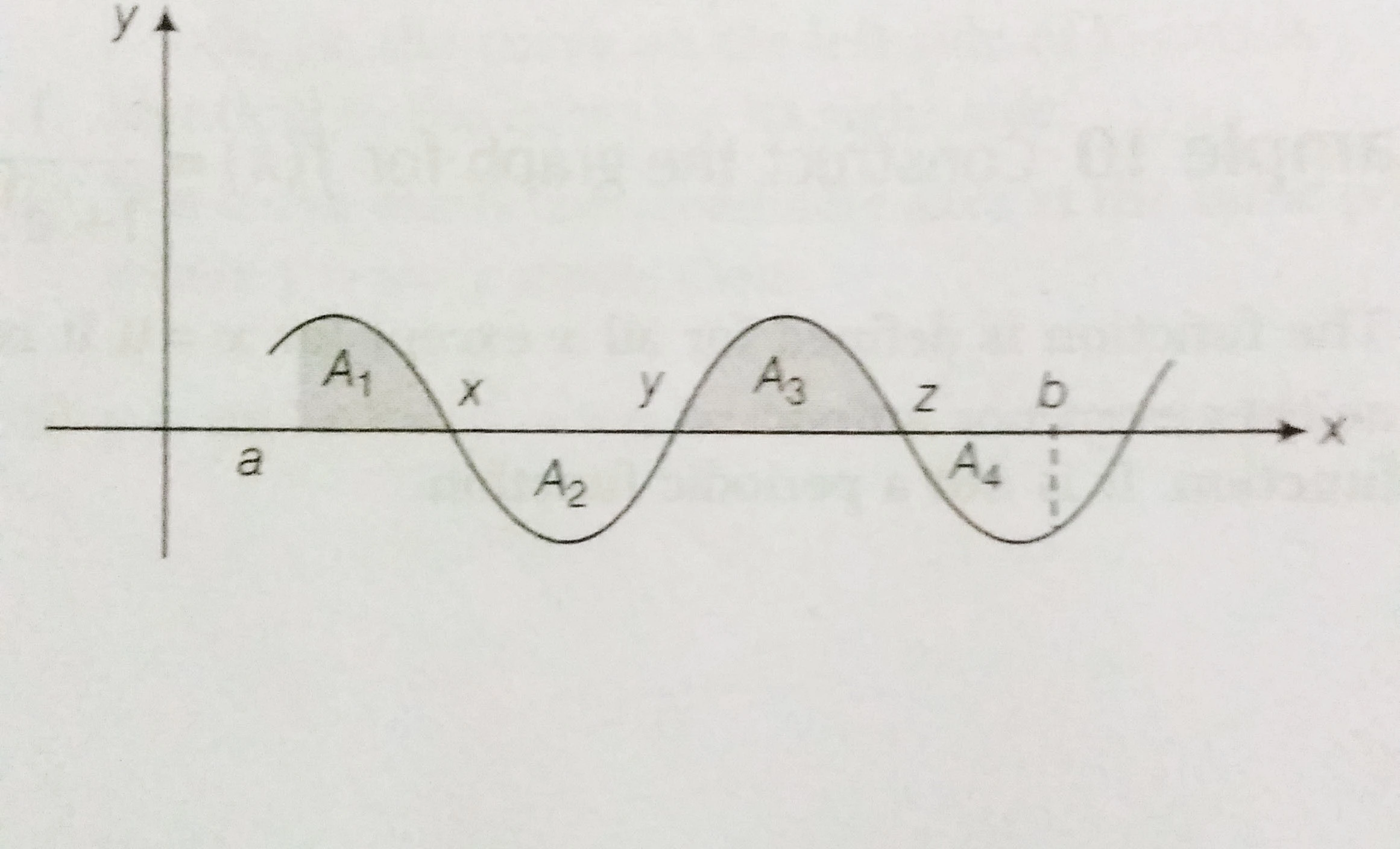Then ba f(x) dx will equal A1 -A2 +A3-A4 and not A1 +A2 +A3+ A4

If we need to evaluate   A1 +A2 +A3+ A4,

Calculate, xa f(x)dx+|yx f(x)dx|+ |bz f(x)dx]

From this, it should be also obvious that

|abf(x) dx |≤∫ba|f(x)|dx

1. The area under the curve y=f(x) from x=a to x =b is equal in magnitude but opposite in sign to the area under the same curve from

x=b to x=a that is

ab f(x) dx= - abf(x) dx

This property is obvious if you consider the Newton Leibnitz Formula. If g(x) is the anti-derivative of

x(f), then ba f(x) dx is g(b) – g(a) while ba f(x)dx is g(a) – g(b)

1. The area under the curve y=f(x) from x=a to x=b can be written as the sum of the area under the curve from x=a to x=c and from x=c to x=b ,

ba f(x) dx=ca(x)dx + bc f(x) dx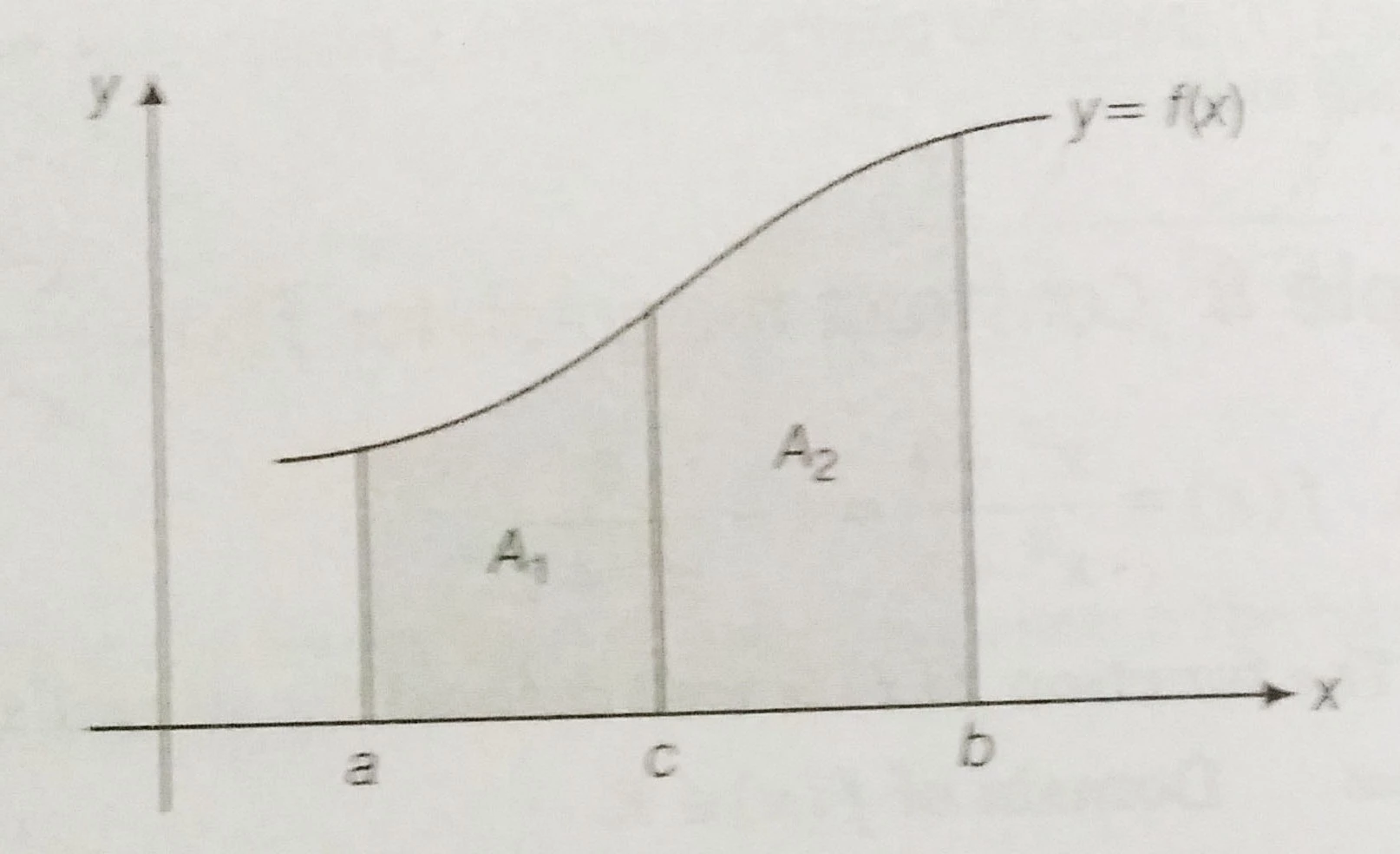Let us consider an example, let cϵ(a,b)

it can be seen that the area under the curve from x=a to x=b is

A  is A1+A2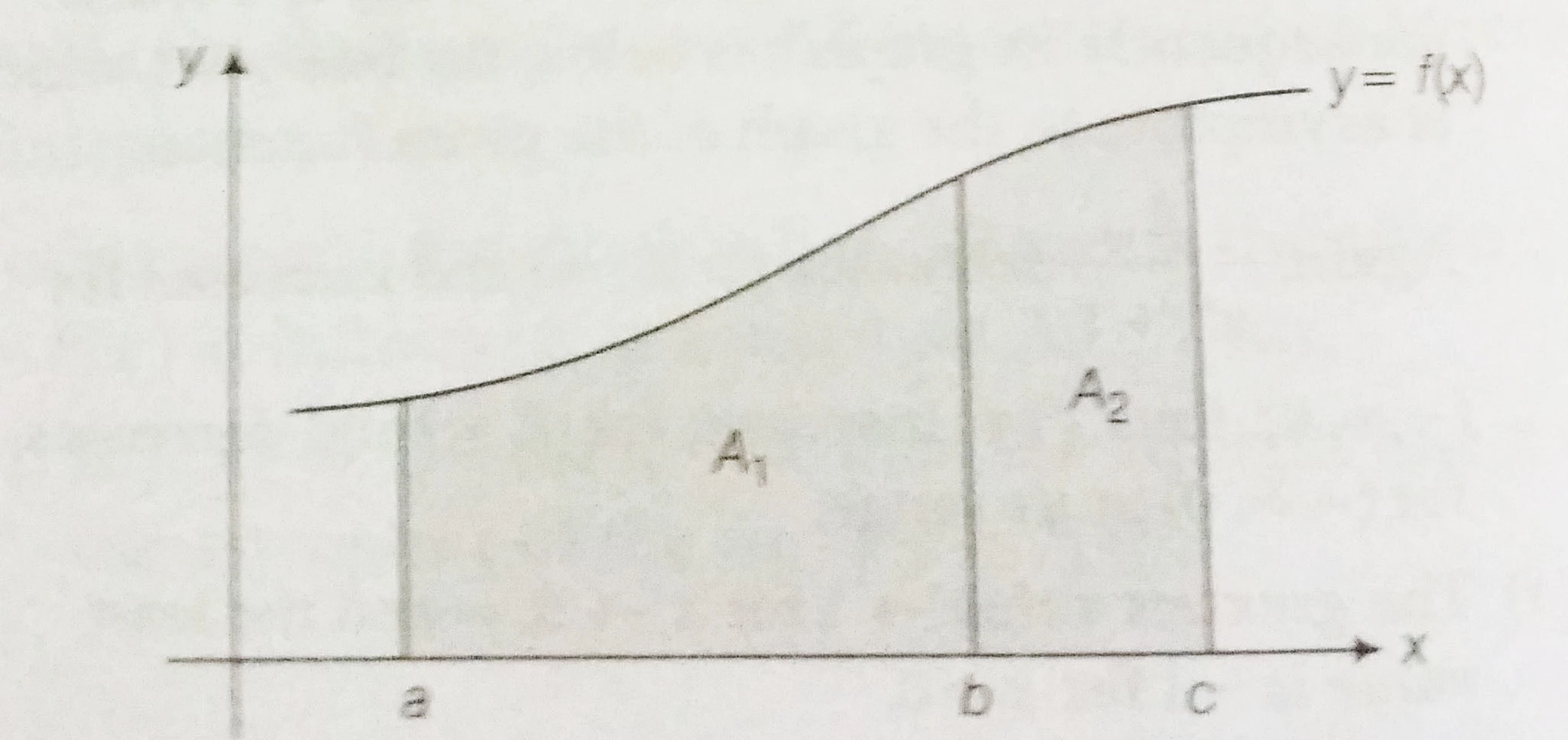Note that c need not lie between a and b for this relation to hold true. Suppose that c>b

Observe that A = ba f(x) dx = (A+A1) – A1

=ca f(x) dx - ∫cb f(x) dx

=ca f(x)dx +∫ba f(x) dx

Analytically, this relation can be proved easily using the Newton Leibnitz formula.

1. Let f(x) > g(x) on the interval [a, b]. then,

ba f(x) dx >∫ba g(x) dx

This is because the curve of f(x) lies above the curve of g(x), or equivalently, the curve of f(x)-g(x) lies above the x-axis for [a. b]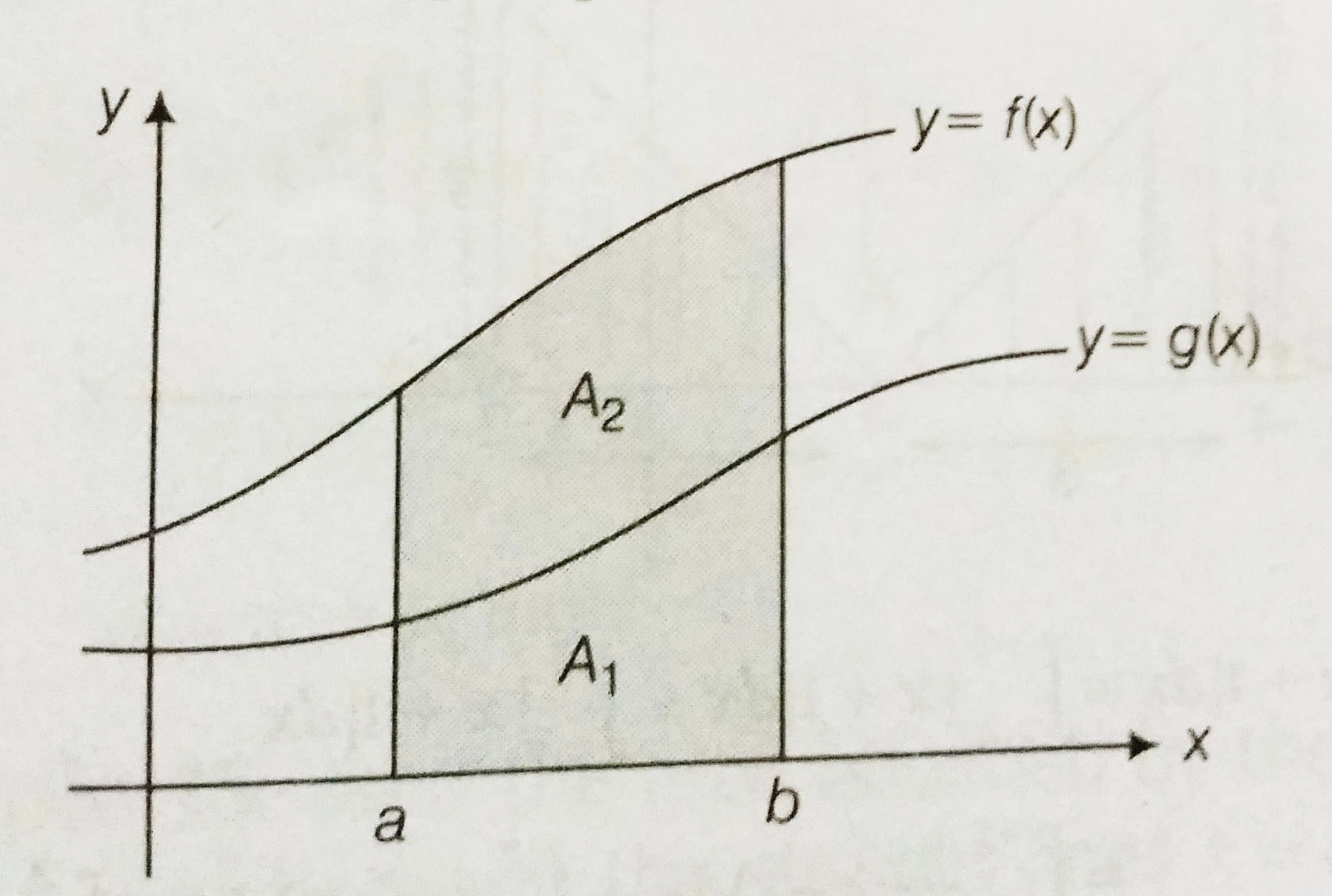This is an example where f(x)>g(x)>0

ba f(x) dx= A1 + A2

While ba g(x)dx = A1

Similarly, if f(x) < g(x) on the interval [a, b], then

ba f(x) dx <bag(x) dx

1. Let us consider the integral of f1 (x) + f2(x) from x=a to evaluate the are under f1 (x) + f2 (x) , we can separately evaluate the area under f1(x) and the area under f2(x) and add the two area

Thus,

ba f1(x)+f2 (x)) dx = ∫ba f1(x)dx+∫ba f2 (x)dx

Now consider the integral and then multiply it by k that is of kf(x) from x=a to x= b.

To evaluate the area under kf (x), we can first evaluate the area under f(x)

ba kf (x) dx = kba f(x)dx.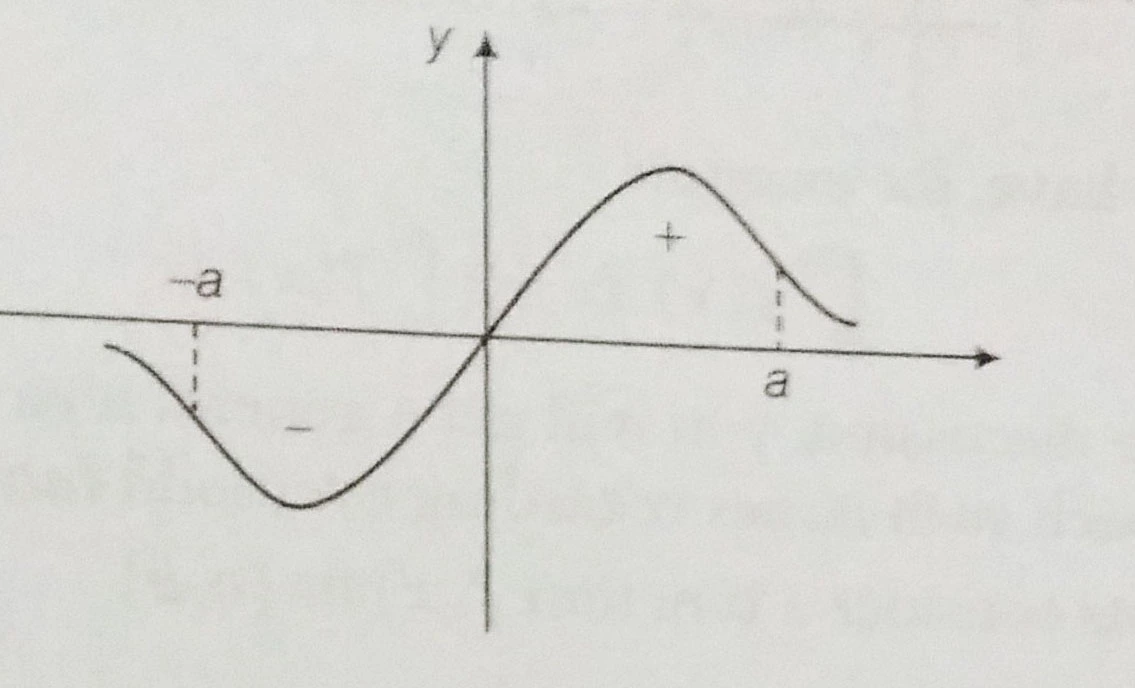1. Consider the odd function f(x), that is f(x) = -f(-x). This means that the graph of f(x) is symmetric about the origin.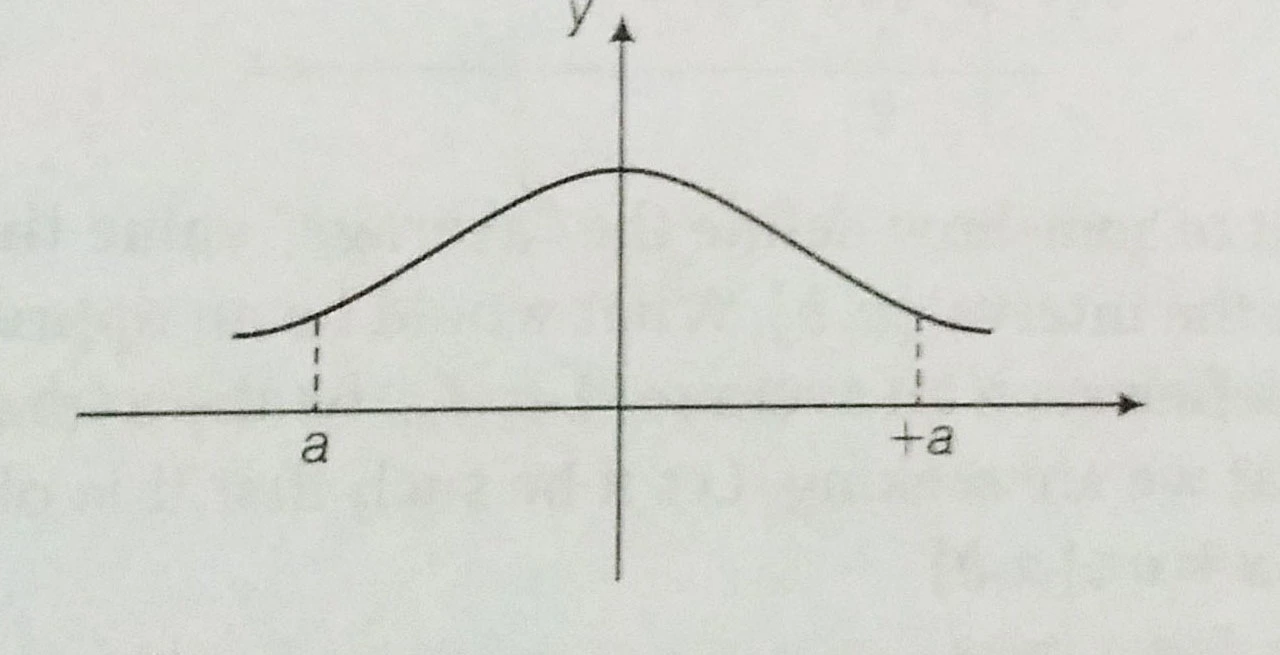From the figure, it should be obvious that a-a f(x0 dx = 0

Because the area on the left side and that on the right algebraically add to 0

Similarly, if f(x) was even, that is f(x) = f(-x)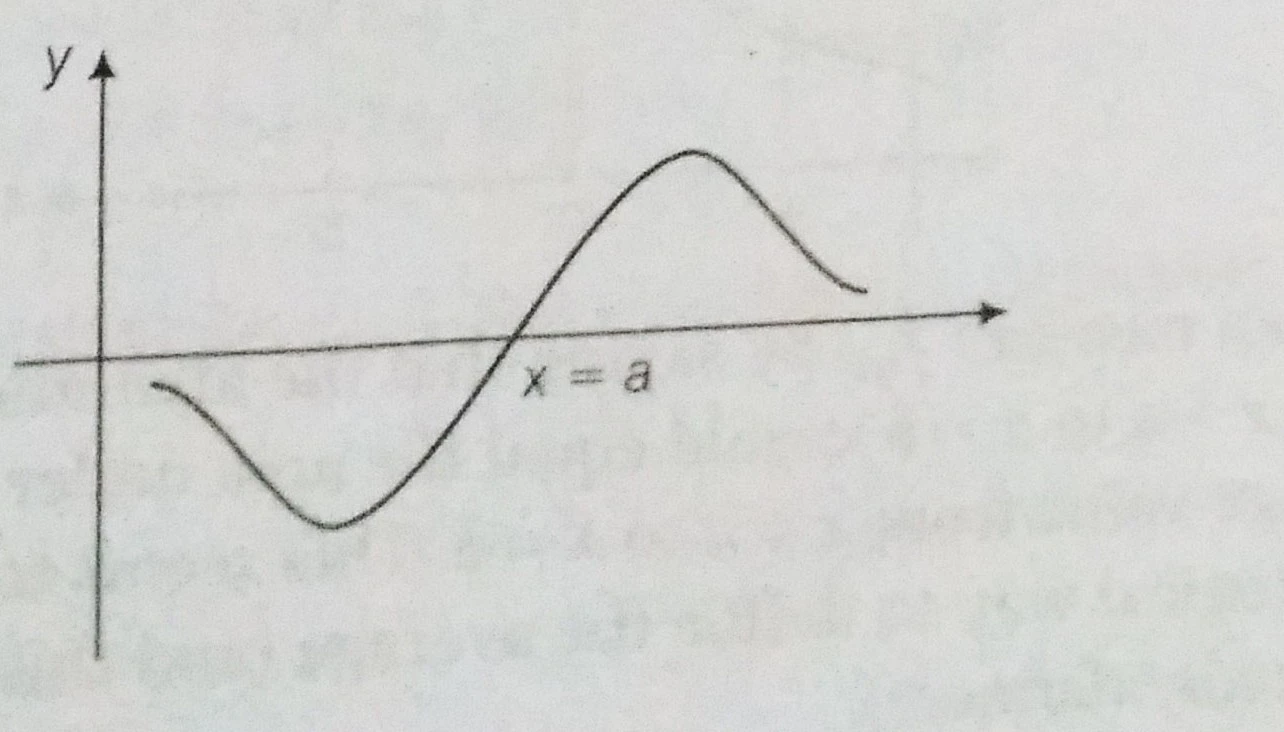a-a f(x) dx = 2a0f(x) dx because the graph is symmetrical about the y-axis. If you recall the discussion in the unit on functions, a function can also be even or odd about any arbitrary point x=a. Let us suppose that f(x) is odd about x= a, that is f(x) = -f(2a-x)

The points x and 2a-x lie equidistant from x=a on either side of it.

Suppose for example, that we need to calculate 2aa  .f(x) dx

It is obvious that this will be 0 since we are considering an equal variation on either side of x = a the area x=0 to x=a and the area from x=a to x=2a will add algebraically to 0.

Similarly, if f(x) is even about x=a that is f(x) = f(2a-x)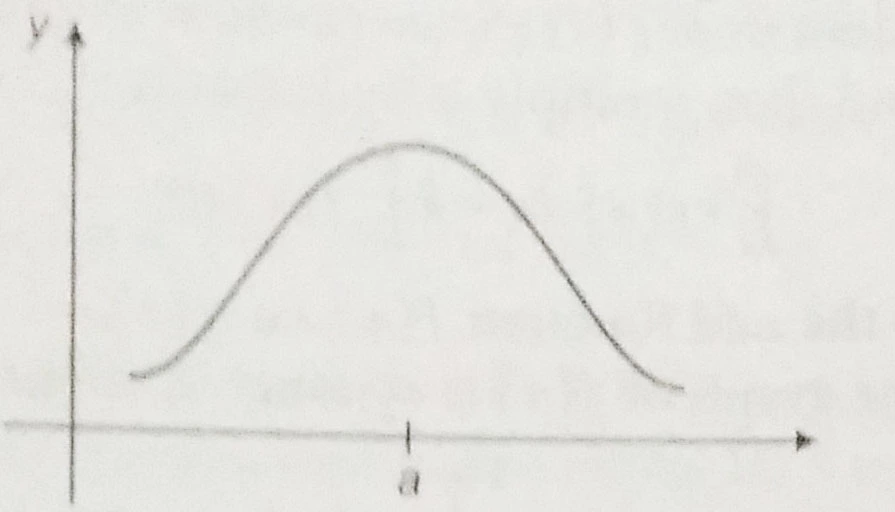Then we have, for example

2a0f(x) dx =2 a0 f(x) dx

From the discussion, you will get a general idea as to how to approach such issues-even/odd functions.

1. Let us discuss a function f(x) on [a, b]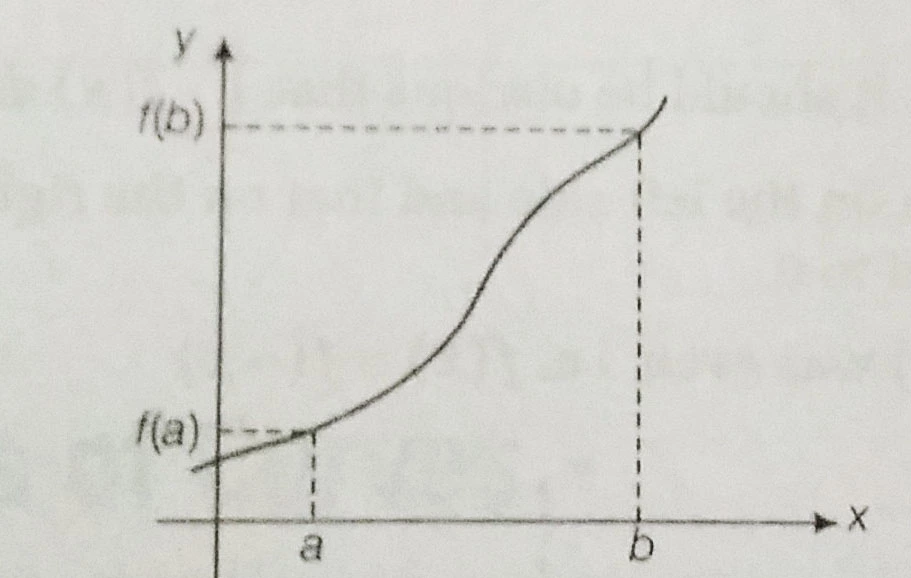We went to define the average value that f(x) takes on the interval [a, b]. Let fav be the average value that we are seeking. Let it be such that it is obtained at some x=cϵ[a, b].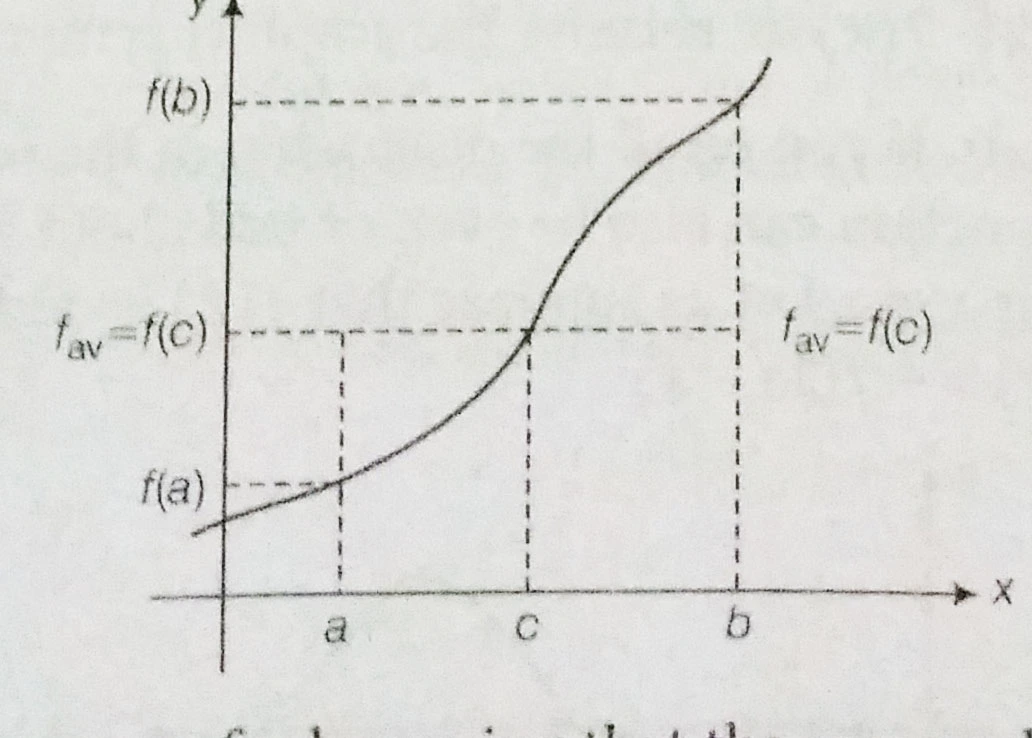We can measure fav by saying that the area under f(x) from x=a to x=b should equal the area under the average value from x=a to x=b. This seems to be the only logical way to define the average (and this is how it is actually defined).

Thus fav(b-a) = ba f(x)dx fav = 1/b-aba f(x0 dx.

This value is attained for at least one c ϵ (a, b) (under the constraint that f is continuous, of course).

### Area Between Two Curves

To find the area between two or more curves, for instance, we can learn to calculate that through cases:

1. If f and h are functions of x such that f(x) h(x) for all x in the intervals [x1,x2] the shaded area is given by

Area = x2x1(f(x)-h(x))dx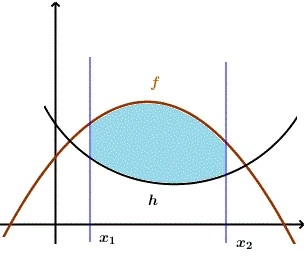1. If z and x are functions of y such that z(y)x(y) for all the y in the interval[y1,y2] the area of the shaded region.

Area = y2y1  (Z(y)-X(y)) dy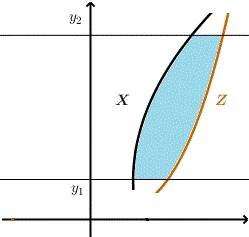## Significance of Doubtnut and its Benefits

1. Doubtnut is an online tutoring platform provides NCERT solutions that can easily be accessed by anyone at zero cost.
2. The main motive of our website is to provide knowledge and the best learning material for each and every student so that they can prepare well for their exams.
3. At Doubtnut, we focus on making the content easily understandable with the use of video tutorials and graphics so that students find it interesting and develop an interest in their studies.
4. For any queries and doubts, students can click a photo of their doubts and post it on the website or the playstore app of the doubtnut. The solution will be provided swiftly.
5. Doubtnut is the best platform for students for self-study purposes.  It helps students to practice exercises and questions by providing solutions for the same in the comfort of their own home.